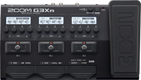# Lofi FUZZ- FIZZ

Discussion in 'Zoom G3n/G3Xn/G5n' started by D I Y - R, Jan 18, 2022.

1. Lofi FUZZ- FIZZDevice: Zoom G3Xn
Firmware: 2.20

Name on device: Lofi FUZZ
Optimized for: Phones/Speaker

Effects chain:Direct Record. lofi Fuzz - Fizz.
a Patch I Use for recording Lofi Fuzzy fizz guitar. & delay for extra Depth

Effect: "TS Drive" (Overdrive / Distortion), active - "yes"
"Gain" = 30
"Boost" = Off
"Tone" = 25
"Volume" = 64

Effect: "XtasyBlue" (Amp simulator), active - "yes"
"Bass" = 51
"Middle" = 52
"Treble" = 44
"Presence" = 30
"STRCT" = HI
"Gain" = 60
"Volume" = 50
"SOLO" = 1

Effect: "BGN4x12" (Cabinet), active - "yes"
"MIC" = ON
"D57: D421" = 40
"Hi" = 70
"Lo" = 65

Effect: "Gt GEQ 7" (Filter), active - "yes"
"100 Hz" = -7.0
"200 Hz" = -7.5
"400 Hz" = -6.5
"800 Hz" = -12.0
"1.6 kHz" = 0.0
"3.2 kHz" = 12.0
"6.4 kHz" = 12.0
"Volume" = 40
"100 Hz" = 0.0
"200 Hz" = 0.0
"400 Hz" = 0.0
"800 Hz" = 0.0
"1.6 kHz" = 0.0
"3.2 kHz" = 0.0
"6.4 kHz" = 0.0
"Volume" = 80
"Parameter set" = A

Effect: "P-P Delay" (Delay), active - "no"
"Time" = Quarter
"F.B." = 61
"Mix" = 45
"Tail" = Off
Patch Volume: 41